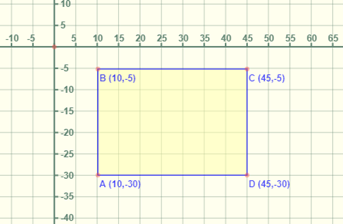Mathematics
Easy

Question

# Find the perimeter of the rectangle whose coordinates are as follows## 110    120    825    875Hint:

## The correct answer is: 120

### The coordinates of the rectangle ABCD areA is (10,-30)B is (10,-5)C is (45,-5)D is (45,-30)We have to find the length of the adjacent sides.To find the length we will use the following criteria:1) If x coordinate is same, we will find the absolute distance using y coordinate. An absolute distance is the length of the point from the respective axis. If x coordinate is same then its length is measured from x axis. It is a positive quantity.2) If y coordinate is same we will use the x coordinate to find absolute distance.3) As the points are in same quadrant, we will have to subtract the absolute distances to find length.We will consider the side AB as length and BC as breadth.We will find the length of side AB.For the points A and B, x coordinate is same. We will find the absolute distance using y coordinate. It is distance from x axis.Absolute distance of the point A = |-30|Absolute distance of the point B = |-5|Length of AB = |-30| - |-5|= 30 – 5= 25Length of the rectangle is 25 units.We will find the length of side BC.For the points B and C, y coordinate is same. We will find the absolute distance using x coordinate. It is distance from y axis.Absolute distance of the point B = |10|Absolute distance of the point C = |45|Length of BC = |45| - |10|= 45 - 10= 35Breadth of the rectangle is 35 units.The formula for area is 2(length + breadth)Area of rectangle ABCD = 2(length + breadth)= 2(25 + 35)= 120 unitsTherefore, area of the rectangle is 120 units.

For such questions, we have to see if the points of the rectangle are in same quadrant or adjacent quadrants. For points in adjacent quadrants, we have to add the absolute values. We can use distance formula to find the length of sides of rectangle too.

### Related Questions to study#### With Turito Foundation.#### Get an Expert Advice From Turito.